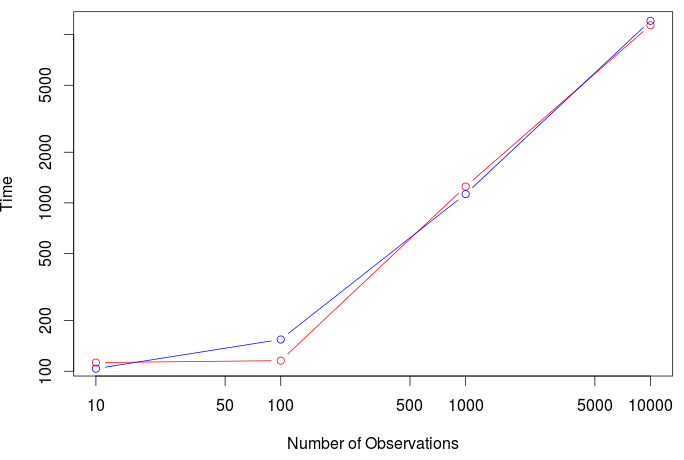Want to share your content on R-bloggers? click here if you have a blog, or here if you don't.

This afternoon, while I was discussing with Montserrat (aka @mguillen_estany) we were wondering how long it might take to run a regression model. More specifically, how long it might take if we use a Bayesian approach. My guess was that the time should probably be linear in , the number of observations. But I thought I would be good to check.

Let us generate a big dataset, with one million rows,

```> n=1e6
> X=runif(n)
> Y=2+5*X+rnorm(n)
> B=data.frame(X,Y)```

Consider as a benchmark the standard linear regression,

```> lm_freq = function(n){
+   idx = sample(1:1e6,size=n)
+   reg = lm(Y~X,data=B[idx,])
+   summary(reg)
+ }```

Here the regression is a subset of smaller size. We can do the same with a Bayesian approach, using stan,

```> stan_lm ="
+ data {
+ int N;
+ vector[N] x;
+ vector[N] y;
+ }
+ parameters {
+ real alpha;
+ real beta;
+ real tau;
+ }
+ transformed parameters {
+ real sigma;
+ sigma <- 1 / sqrt(tau);
+ }
+ model{
+ y ~ normal(alpha + beta * x, sigma);
+ alpha ~ normal(0, 10);
+ beta ~ normal(0, 10);
+ tau ~ gamma(0.001, 0.001);
+ }
+ "```

Define then the model

```> library(rstan)
> system.time(
stanmodel <<- stan_model(model_code = stan_lm))
utilisateur     système      écoulé
0.043       0.000       0.043```

We want to see how long it might take to run a regression,

```> lm_bayes = function(n){
+   idx = sample(1:1e6,size=n)
+   fit = sampling(stanmodel,
+       data = list(N=n,
+                   x=X[idx],
+                   y=Y[idx]),
+       iter = 1000, warmup=200)
+   summary(fit)
+ }```

We use the following package to see how long it takes

```> library(microbenchmark)
> time_lm = function(n){
+  M = microbenchmark(lm_freq(n),
+      lm_bayes(n),times=50)
+  return(apply( matrix(M\$time,nrow=2),1,mean))
+ }```

We can now compare the time it took with ten, one hundred, on thousand, and ten thousand observations,

```> vN = c(10,100,1000,10000)
> T = Vectorize(time_lm)(vN)```

we can then plot it

```> plot(vN,T[2,]/1e6,log="xy",col="red",type="b",
+      xlab="Number of Observations",ylab="Time")
> lines(vN,T[1,]/1e6,col="blue",type="b")```It looks like (if we forget about the very small sample) that the time it takes to run a regression is linear, with the two techniques (the frequentist and the Bayesian ones).

And actually, the same story olds for logistic regressions. Consider the following dataset

```> n=1e6
> X=runif(n)
> S=-3+2*X+rnorm(n)
> Y=rbinom(n,size=1,prob=exp(S)/(1+exp(S)))
> B=data.frame(X,Y)```

The frequentist version of the logistic regression is

```> glm_freq = function(n){
+   idx = sample(1:1e6,size=n)
+   reg = glm(Y~X,data=B[idx,],family=binomial)
+   summary(reg)
+ }```

and the Bayesian one, using stan,

```> stan_glm = "
+ data {
+ int N;
+ vector[N] x;
+ int<lower=0,upper=1> y[N];
+ }
+ parameters {
+ real alpha;
+ real beta;
+ }
+ model {
+ alpha ~ normal(0, 10);
+ beta ~ normal(0, 10);
+ y ~ bernoulli_logit(alpha + beta * x);
+ }
+ "
> stanmodel = stan_model(model_code = stan_glm) )
> glm_bayes = function(n){
+   idx = sample(1:1e6,size=n)
+   fit = sampling(stanmodel,
+        data = list(N=n,
+        x = X[idx],
+        y = Y[idx]),
+        iter = 1000, warmup=200)
+   summary(fit)
+ }```

Again, we can see how long it takes to run those regression models

```> time_gl m= function(n){
+   M = microbenchmark(glm_freq(n),
+   glm_bayes(n),times=50)
+   return(apply( matrix(M\$time,nrow=2),1,mean))
+ }```